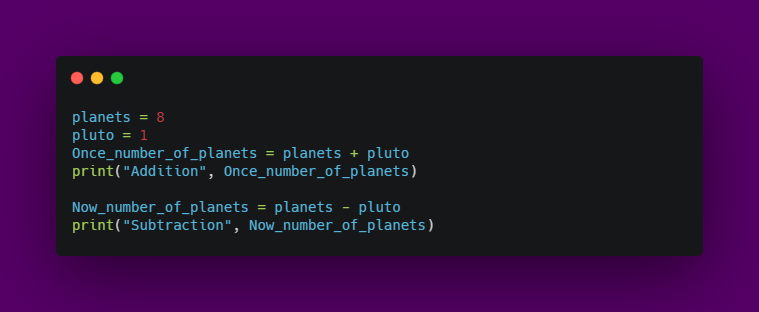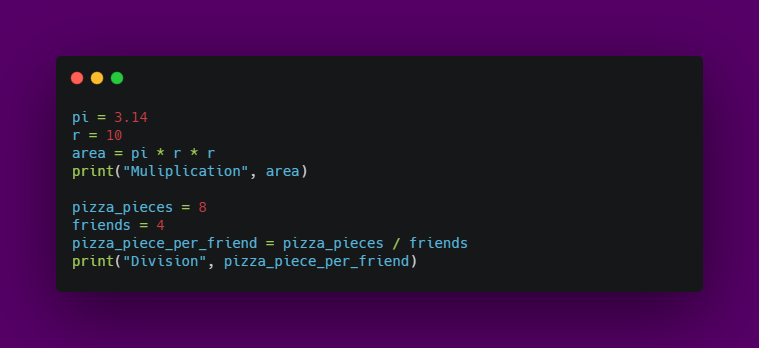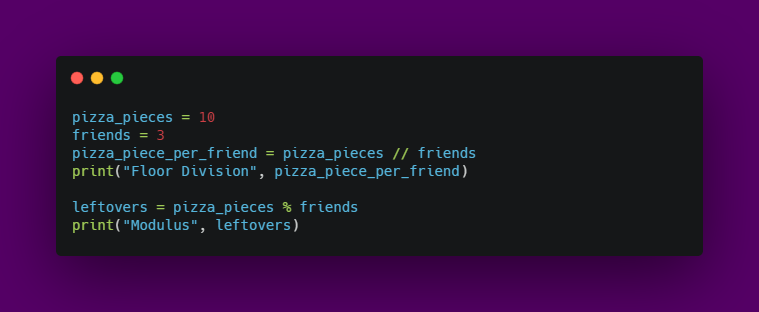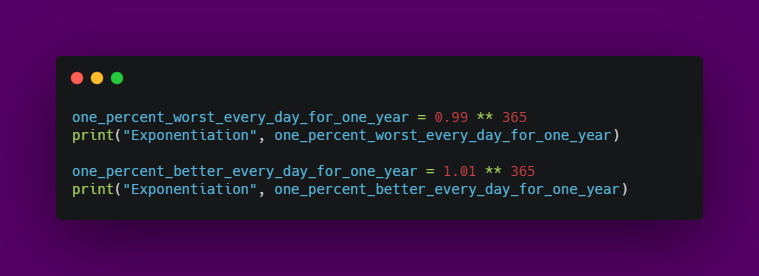Published on

# Arithmetic operators in python

Authors
•Name
Aswin Barath
@AswinBarath2

Operators are used to performing operations on any given data stored inside variables.

And arithmetic operators makes mathematical operations possible on operands in a program.

I know! I know! This is a basic concept! But let's make it fun!

## Starting with addition and subtractionOutput:

``````Addition 9
Subtraction 8
``````

## Now, Multiplication and DivisionOutput:

``````Multiplication 314.0
Division 2.0
``````

## Special arithmetic operators

• Floor division - `//`: rounds of the result to the nearest whole number.
• Modulus - `%`: produces the remainder of the numbersOutput:
``````Floor Division 3
Modulus 1
``````
• Exponentiation - ** : produces the power of given numbersOutput:
``````Exponentiation 0.025517964452291125
Exponentiation 37.78343433288728
``````